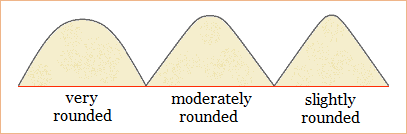# Volume of a Pile of Gravel or Sand

Gravel Volume Calculator
Height    inches
Base Rectagular Base
Width = inches
Length = inches

Circular Base
Peak Slightly Rounded
Moderately Rounded
Very Rounded

The volume of a pile of sand or gravel depends on the dimensions and shape of the pile. For conical and pyramid-shaped piles of gravel, you can use the cone and pyramid volume formulas to compute a rough estimate. You can then adjust this estimate according to how rounded the top of the cone or pyramid is.

### Cone Volume

The formula for the volume of a cone is πR²H/3, where R is the radius of the circular base and H is the height of the cone. An alternate version of the formula is πD²H/12, where D is the diameter.

### Pyramid Volume

The volume of a pyramid with a rectangular base is LWH/3, where L is the length, W is the width, and H is the height.

### How a Rounded Peak Affects the Volume

When the peak of the pile of gravel or sand is rounded, there is extra volume near the top. For slightly rounded tops, the volume may be increased by a factor of 2% to 5%. If the pile has a moderately rounded peak, the volume may be increased by 15% to 20%. And for very rounded tops, the volume may increase by 30% to 40%.Example Calculation: Suppose a pile of gravel is in the shape of a cone with a height of 24 inches and a diameter of 40 inches. The top of the cone is moderately rounded.

First, find the basic conical volume in cubic inches by using the formula πD²H/12. This gives you

π(40)²(24)/12 = 10053 cubic inches.

Next, convert this to cubic yards by dividing by 46656. This gives you 10053/46656 = 0.2155 cubic yards. To account for the moderately rounded peak, add 20% to this figure. The final answer is approximately

(0.2155)(1.2) = 0.2586 cubic yards.